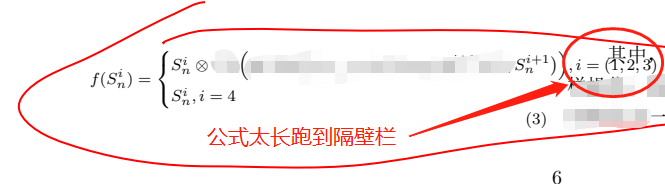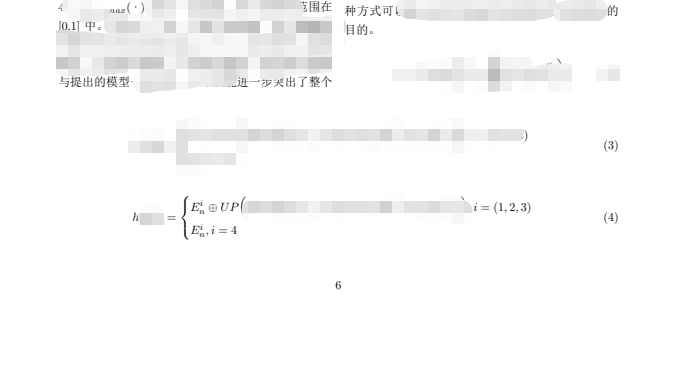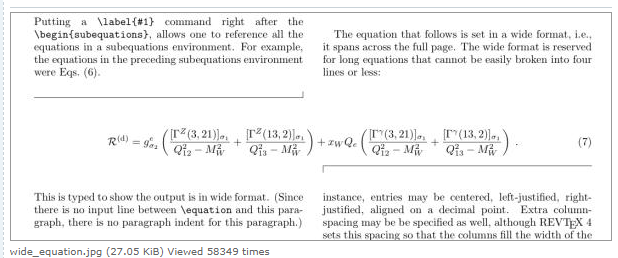• latex双栏排版公式太长
千次阅读
2020-07-02 16:42:59

转自https://blog.csdn.net/lma_hzq/article/details/104438668
1.导入宏包\usepackage{stfloats}。
2.

\newcounter{TempEqCnt} % 创建临时变量TempEqCnt
\setcounter{TempEqCnt}{\value{equation}} % 将当前公式序号 赋给TempEqCnt
\setcounter{equation}{15} % 当前公式序号变为x，x等于长公式应有的序号减1.
\begin{figure*}[ht] %hb代表放在文章底部，%ht为放在文章顶部
\begin{equation}
公式（16）
\end{equation}
\begin{equation}
公式（17）
\end{equation}
\begin{equation}
公式（18）
\end{equation}
\end{figure*}


3.注意事项：公式会跑到文章的后一页，要想放在当前页需要在上一页代码处进行插入，但是这么操作后面的公式序号会乱，需要自定义后面公式的序号。

更多相关内容
• 中，有一道公式长度实在太长，左思右想，发现无非几种解决办法 方案 1、缩小公式字体 办法是直接 用\begin{footnotesize} \begin{footnotesize} #设置字体大小 可以选择small tiny \begin{...

目录

问题

方案

1、缩小公式字体

2、公式内容换行

3、公式跨栏居中显示

这些方法我摸索了一个早上才找到的，如果对大家有帮助，请大家给我一键三连！！非常感谢！！！

# 问题

在双列栏中，有一道公式长度实在太长，左思右想，发现无非几种解决办法# 方案

## 1、缩小公式字体

办法是直接 用 \begin{footnotesize}

\begin{footnotesize}  #设置字体大小  可以选择small  tiny
\begin{equation}
\begin{aligned}
S'_{i} =  S_{i} \otimes FP_{i}  + S_{i} + FP_{i}
\end{aligned}
\label{eq_s}
\end{equation}
\end{footnotesize}


## 2、公式内容换行

换行比较简单 直接 \\ 换行，但是很多情况下并不合适

## 3、公式跨栏居中显示

### 方案一

网上一搜几乎这种答案，但是解决不了我的问题

\onecolumn 暂时切换单栏,输入公式,在 \twocolumn 切换回双栏。 或者用 multicol 宏包, 用 \begin{multicols}{2} 这种方法切换单双栏。 我记得这两种办法会导致一个页眉章节码取右不取左的小bug,有一个宏包是来搞定这个bug的,但是名字我给忘了。。 总之我看到的大部分都是这么做的, IEEE甚至那个什么都这么做,

使用\onecolumn 暂时切换单栏,输入公式,在 \twocolumn 切换回双栏。 会出现你的公式变成在单独一页上面显示

使用用 multicol 宏包, 用 \begin{multicols}{2} 这种方法切换单双栏。 由于我一开始就是 双栏，这种办法的结果就是在我双栏中的一栏再去分双栏，然后公式还是在原来的一栏里面显示，这就是跨栏，但由于我是用\documentclass[10pt, twocolumn]{article} 分栏的，所以这种并不适合我。

### 方案二

效果图思路

1、公式跨栏居中显示，\begin{equation }没有支持居中显示（加了一个 * 没起作用）  \begin{figure}可以加上一个 * 就可以居中显示，是否可以借鉴呢？

2、使用\begin{figure}，在figure里面写equation，利用\begin{figure*}使得我们的公式居中。这样子写的话，也没有公式的编号问题，非常方便直接


\begin{figure*}[hb] %hb代表放在文章底部，%ht为放在文章顶部
\centering
\begin{equation}
f(S_{n}^i)=
\begin{cases}
S_{n}^i\otimes S_{n}^{i+1},i=(1,2,3)\\
S_{n}^i,i=4
\end{cases}
\label{eq_h_s}
\end{equation}

\end{figure*}

# 这个方法我摸索了一个早上才找到的，如果对大家有帮助，请大家给我一键三连！！非常感谢！！！

展开全文• Elsevier 双栏模板下的长公式 \documentclass[5p,preprint,12pt]{elsarticle} \usepackage{amsmath} \usepackage{mathtools, cuted} \usepackage[english]{babel} \usepackage{lipsum} \begin{document} \lipsum[1-2...

Elsevier 双栏模板下的长公式

\documentclass[5p,preprint,12pt]{elsarticle}
\usepackage{amsmath}
\usepackage{mathtools, cuted}
\usepackage[english]{babel}
\usepackage{lipsum}
\begin{document}

\lipsum[1-2]
\begin{strip}
\begin{equation}
\sum_{q_\mathrm{tot}=0}^{Q_A+Q_B-2}\sum_{q_A=0}^{q_\mathrm{tot}}{\textstyle\binom{Q_A-1}{q_A}\binom{Q_B-1}{q_B}}x^{q_\mathrm{tot}},
\end{equation}
\end{strip}
\lipsum[1-3]

\end{document}

### 原文：long equation across whole page in two-column document

\documentclass{article}
\usepackage[margin=3cm]{geometry}
\usepackage{lipsum,amsmath,multicol}
\begin{document}

\begin{multicols}{2}
\lipsum[1-3]
\end{multicols}
\par\noindent\rule{\dimexpr(0.5\textwidth-0.5\columnsep-0.4pt)}{0.4pt}%
\rule{0.4pt}{6pt}

\begin{equation}
\mathcal{R}^{(\text{d})}=
g_{\sigma_2}^e
\left(
\frac{[\Gamma^Z(3,21)]_{\sigma_1}}{Q_{12}^2-M_W^2}
+\frac{[\Gamma^Z(13,2)]_{\sigma_1}}{Q_{13}^2-M_W^2}
\right)
+ x_WQ_e
\left(
\frac{[\Gamma^\gamma(3,21)]_{\sigma_1}}{Q_{12}^2-M_W^2}
+\frac{[\Gamma^\gamma(13,2)]_{\sigma_1}}{Q_{13}^2-M_W^2}
\right)\;. \label{eq:wideeq}
\end{equation}

\vspace{\belowdisplayskip}\hfill\rule[-6pt]{0.4pt}{6.4pt}%
\rule{\dimexpr(0.5\textwidth-0.5\columnsep-1pt)}{0.4pt}
\begin{multicols}{2}
\lipsum[4-5]
\end{multicols}

\end{document}参考资料

展开全文• 方法1: IEEE官方修改（本文推荐） \begin{figure*} \begin{equation} \label{eq-13} +++++++++++++++++ Put equation here +++++++++++++++++ \end{equation} \hrulefill % \vspace*{4pt} ...
• 方法1: IEEE官方修改（本文推荐）
\begin{figure*}
\begin{equation}
\label{eq-13}
+++++++++++++++++
Put equation here
+++++++++++++++++
\end{equation}
\hrulefill
% \vspace*{4pt}
\end{figure*}

• 方法2: IEEE官方，\begin{document}前插入\newcounter{mytempeqncnt}
\begin{figure*}[!t]
% ensure that we have normalsize text
\normalsize
% Store the current equation number.
\setcounter{mytempeqncnt}{\value{equation}}
% Set the equation number to one less than the one
% desired for the first equation here.
% The value here will have to changed if equations
% are added or removed prior to the place these
% equations are referenced in the main text.
\setcounter{equation}{12}
\begin{equation}
\label{eqn_dbl_x}

+++++++++++++++++
Put equation here
+++++++++++++++++

\end{equation}
% Restore the current equation number.
\setcounter{equation}{\value{mytempeqncnt}}
% IEEE uses as a separator
\hrulefill
% The spacer can be tweaked to stop underfull vboxes.
\vspace*{4pt}
\end{figure*}

• 方法2：\begin{document}前插入\usepackage{cuted}
\begin{strip}
\begin{equation}
+++++++++++++++++
Put equation here
+++++++++++++++++
\end{strip}

展开全文• 在写论文的时候，发现如果写双栏论文，公式的时候，会影响到另一的显示 ，即会出现以下尴尬情况： 为了解决这个问题，尝试了两种方法，记录如下： 1.对单个公式进行处理，利用LaTeX中的空格进行微调，这样...缩放
• latex双栏模板中使用 通栏的公式或者图 通栏的图 在原来插图的环境中的 figure后加*；具体如下 \begin{figure*}[ht] \label{fig1} \centering \includegraphics[width = 3in]{Fig1.pdf} \caption{The ...
• 带星号的浮动体figure和table环境可以在双栏模板中使用，例如我们以IEEEtran为例，插入通栏公式 \documentclass{IEEEtran} \usepackage{amsmath，lipsum} \begin{document} \lipsum \begin{figure*} \begin{align...
• LaTeX中最实用的双栏转单栏公式表格插入插入公式插入表格 插入公式 \begin{figure*}[b] {\noindent} \rule[-10pt]{18.07cm}{0.1em} \begin{equation} e^{i\pi}+1=0 \end{equation} \end{figure*} ...
• LaTeX表格排版的技巧，表格占满单布局宽度，表格占满单布局中两宽度，或者自定义表格宽度(无论是单还是双栏)
• 本文档介绍了如何使用TeX编辑长公式，在latex的出版说明中并未涉及长公式的编写，作者只是提供给大家一下对长公式的基本处理
• Latex中多行数学公式排版方法: 引入amsmath和amssymb宏包： \usepackage{amsmath} \usepackage{amssymb} gather环境实现多行数学公式排版 其中用双反斜\\杠实现换行功能，此环境下不但实现了对公式的分行排版，...
• Latex不止可用来写论文，对教师来说排版试卷也非常好用。 Latex对于生成复杂表格和数学公式，这一点表现得尤为突出。因此它非常适用于生成高印刷质量的科技和数学类文档。这个系统同样适用于生成从简单的信件到完整...
• Latex是一门用于排版的语言，常用于数学建模论文的排版，可用于Typora记录数学公式
• Latex排版数学公式，介绍latex排版数学公式的各种命令，及数学红包，是学习latex编辑数学公式的好教材
• Latex使用技巧：双栏模式下的跨栏长公式（APS期刊,PRL,PRA,PRB…） 此博文包含图片 (2017-03-16 16:41:49)转载▼ 标签： latex ctec 论文写作 技巧 长公式 分类： Latex技巧 在百度上查了半天，几乎所有的方法就是用...傅立叶分析
• 当我们输入的公式时，最容易想到的方法是，在会出现越界的情况，使用强制换行\\，但是这种方法在公式中行不通。 通常，我们行间公式的换行可使用split 环境来实现。如 \[ \begin{split} x &= \...
• 关于的入门，可以参考：《LaTeX环境配置》，和《LaTeX入门(一)》当然，我不打算继续从 入门(二)开始写起，关于这个新的专题：学习指南，将会一直写下去，与 R 语言系列一样不断地更新，同时，我希望，尽可能地解释...
• LaTeX文档的最开始声明使用单双栏可选项： 单 \documentclass[onecolumn]{article} 双栏 \documentclass[twocolumn]{article}macos
• 在mathtype中编辑公式 对Mathtype 进行简单设置 在word中对公式代码进行修改 将公式代码粘贴到latex的编辑软件中 以WinEdt 7.0 为例，直接将在word中修改后的公式代码复制粘贴到latex的正文中， 运行之后就生成的...
• 这个问题集是针对公式排版过程中经常遇到的一些问题而精心制作的。 在制作过程中，我们力图消灭每一个笔误，但是随着编写页码的不断增 ，原文件的维护变得很困难，我们希望各位网友能够对我们所做的工作提 出批评...
• 数学建模不会 LaTex 排版 | 教你如何在 Word 中优雅地使用漂亮的 LaTex 公式
• 我们在论文中常需要加入公式，包括行内公式与行间公式两种。...最简单的LaTeX公式插入的方式是使用mathtype编辑好公式，直接复制到LaTeX里面。首先需要在mathtype里面进行设置（Prferences里面）： ...
• 在mathtype中编辑公式 将mathtype中编辑的公式复制粘贴到word中 对word中的公式进行转换 对公式代码进行修改 将公式代码粘贴到latex的编辑软件中 以WinEdt 7.0 为例，直接将在word中修改后的公式代码复制粘贴到latex...
• latex双栏排版中的图表及公式通栏 \begin{multicols}{2} Bla bla... \end{multicols} % \leftsep \begin{equation} \label{eqn:planar:Hz02} \left \{\begin{array}{l} \delta A + \...
• LaTex 关于优化问题公式排版及算法格式排版例子宏包LaTex公式书写公式对齐alignedsplit算法格式排版模拟退火算法 宏包 写算法别忘记导入算法的宏包： \usepackage{algorithm} \usepackage{algorithmic} LaTex公式...算法
• 需要对齐的长公式可以用split 环境，它本身不能单独使用，因此也称作次环境，必须包含在equation 或其它数学环境内。split 环境用 \ 和& 来分行和设置对齐位置。 \begin{equation} \begin{split} x=&a+b+c...
• 基本的使用包含基本排版流程、常见数学公式、图像绘制和格式调整等基本使用 同时包含Latex的一些高级运用，如文件的拆分，错误处理和合并多个PDF等一些操作， 笔记还包含相关软件的使用，纯文本编辑器和可视化编辑器......